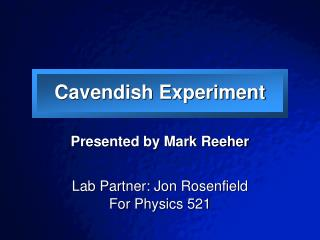DownloadDownload PresentationCavendish Experiment

# Cavendish Experiment

Download Presentation## Cavendish Experiment

- - - - - - - - - - - - - - - - - - - - - - - - - - - E N D - - - - - - - - - - - - - - - - - - - - - - - - - - -
##### Presentation Transcript

1. Cavendish Experiment Presented by Mark Reeher Lab Partner: Jon Rosenfield For Physics 521

2. Presentation Overview • Historical Background • Theory • Experimental Setup and Methods • Results • Analysis of Results • Uncertainties • Conclusions

3. Brief Timeline of Gravitational Physics • 4th Century B.C: Aristotle – tendency of objects to be pulled to Earth • 1645: Ismael Bulliadus - inverse square relation • 1665: Sir Isaac Newton - • 1798: Henry Cavendish – calculation of Universal Gravitation Constant, G • Early 1900s: Einstein- • Inertia-gravitation equivalence • General relativity

4. Cavendish Experiment • John Michell – conception of experiment • Torsion Balance • Henry Cavendish – rebuilt balance and ran experiment in 1797-1798 • Basic Idea – directly measure Fg, find G • Found: G = 6.754 × 10−11 m3kg-1s-2

5. Committee on Data for Science and Technology’s Value

6. Theory – Experimental Design • Large masses brought near small masses • Gravitational force  movement in torsion balance • Study motion to determine Fg • With Fg, measure M, m, r • Newton’s gravitational equation • Result = calculated G

7. Top View Derivation - 1 Fβ Fα

8. Derivation - 2

9. Small Angle Approximation • For simplicity, we assume θ is very small • Torque dot product • Tan θ = θ • This assumption confirmed by finding the largest possible angle of setup • θmax = 0.03884 = 2.226º • ~0.05% difference between tan θ and θ

10. Experimental Setup

11. Experimental Setup Torsion balance enclosure Large masses Vacuum pump (oil) He-Ne laser Ametek plotter (converted)

12. Setup Diagram Laser Plotter

13. Setup Diagram So we need to keep in mind, the plotter reacts to 2θ

14. Fα Fα Fβ Setup Notes • Torsion enclosure pumped to ~100 mTorr • Data recorded automatically in Labview • Photodiode position vs time (4 s intervals) • Six total trials • 2 counter-clockwise (positive) torque • 2 clockwise (negative)torque • 2 no mass

15. Results (Our Measurements) • Given in lab manual • m = 0.019 kg • Mrod = 0.031 kg (square cross section) • L/2 = 15.24 cm • Distance measurements (in inches) • Dd (mirror-diode) = 45 1/32” • ω and θ are found from Matlab data 1 2 4 3

16. Analysis • Data from best fit: • General model: f(x) = a*exp(-x/b)*cos(c*x+d)+e • Coefficients (with 95% confidence bounds):        a =         131  (130.4, 131.6)        b =  1.029e+004  (1.006e+004, 1.051e+004)        c =    0.007577  (0.007575, 0.007579)        d =    0.004448  (0.0001244, 0.008771)        e =       682.1  (681.9, 682.3) • Goodness of fit:   SSE: 1000   R-square: 0.9986   Adjusted R-square: 0.9986   RMSE: 1.002

17. Analysis • I calculation • Κ calculation • Avg K = 2.60588 x 10-7+ 1.197 x 10-11 kg m/s2

18. Analysis • ri calculation (m) • θ calculation • Avg eo from “NM” values: eo = 3.954” + 0.000177” • Define xi = eo - ei

19. Analysis • Now find θ from tan-1: • Finally… we find G (m3s-2): • Avg G = (3.89829 x 10-10+ 1.7129 x 10-11)/M

20. Uncertainty • Total Uncertainty relation for G: 000000000000

21. Uncertainty • Each of the four variables also had combined uncertainty in their calculation • All type A aside from distance measurements • In a few cases, values were averaged:

22. Conclusions • M = 5.701 kg † • Gives us: • GCavendish = 6.754 × 10−11 m3kg-1s-2 • GCODATA = 6.67428 × 10−11 m3kg-1s-2 • Obvious setup interference • MEarth Accepted value = 5.97 x 1024 kg † conversation with Jose